# 运算放大器稳定性分析——稳定性分析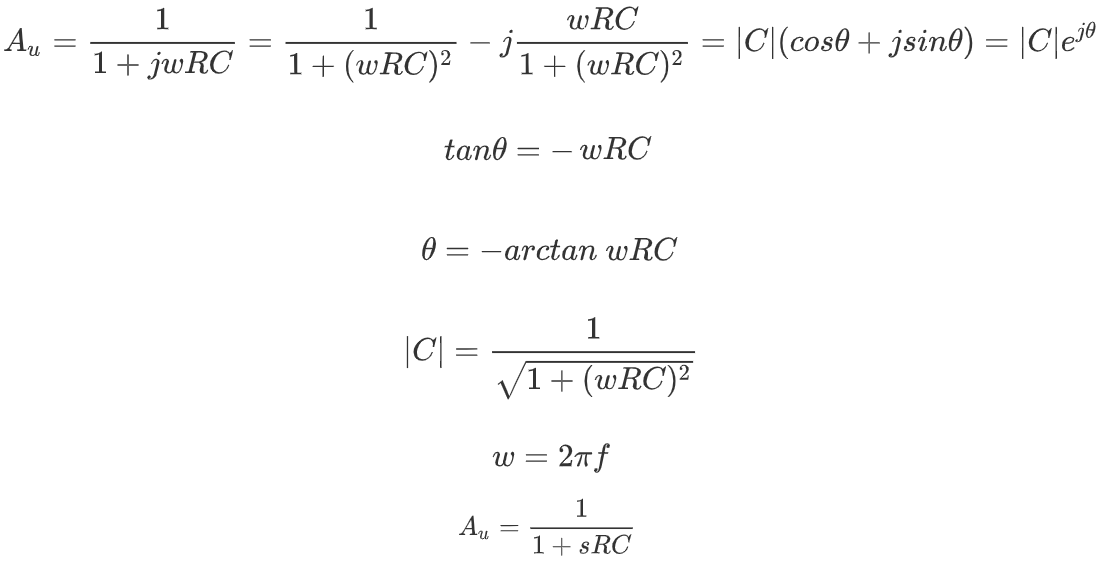s域中定义了零极点，可见有极点，为-1/RC。

• w=0时，|C|=1=0dB，Θ=-arctang(0)=0°

• w=1/RC时，|C|=0.707=-10lg2(dB)=-3dB，即增益降低到0.707倍（即-3dB），Θ=-arctan1=-45°

• w=+∞时，|C|=0=-∞dB。Θ=-arctan(+∞)=-90°

• w=1/10RC，1+(wRC)^2=1.01，则|C|=0.995=-0.043dB，Θ=-arctang(0.1)=-5.7°

• w=1/RC，1+(wRC)^2=2，则|C|=0.707=-3dB，Θ=-arctang(1)=-45°

• w=10/RC，1+(wRC)^2=101，则|C|=0.0995=-20dB，Θ=-arctang(10)=84.3°

• w=100/RC，1+(wRC)^2=10001，则|C|=0.00995=-40dB，Θ=-arctang(100)=89.4°

• w=1000/RC，1+(wRC)^2=1000001，则|C|=0.000995=-60dB，Θ=-arctang(1000)=89.9°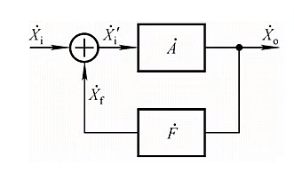反馈模型

A为开环增益，F为反馈增益。求得闭环增益为（假定为负反馈）：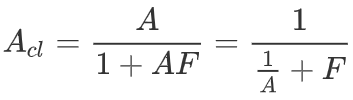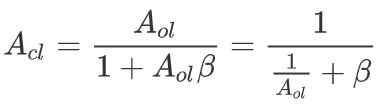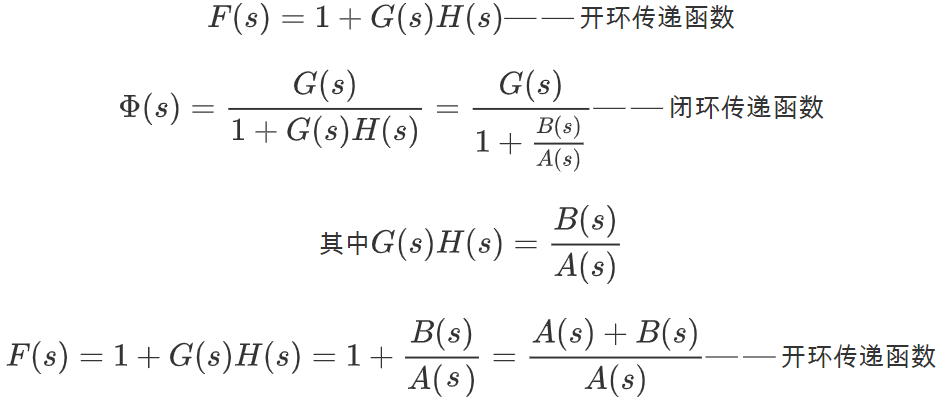Aol为运放本身的增益，可以通过芯片手册上的开环增益曲线估算其Aol(s)，β为反馈增益即输出反馈给输入的信号增益。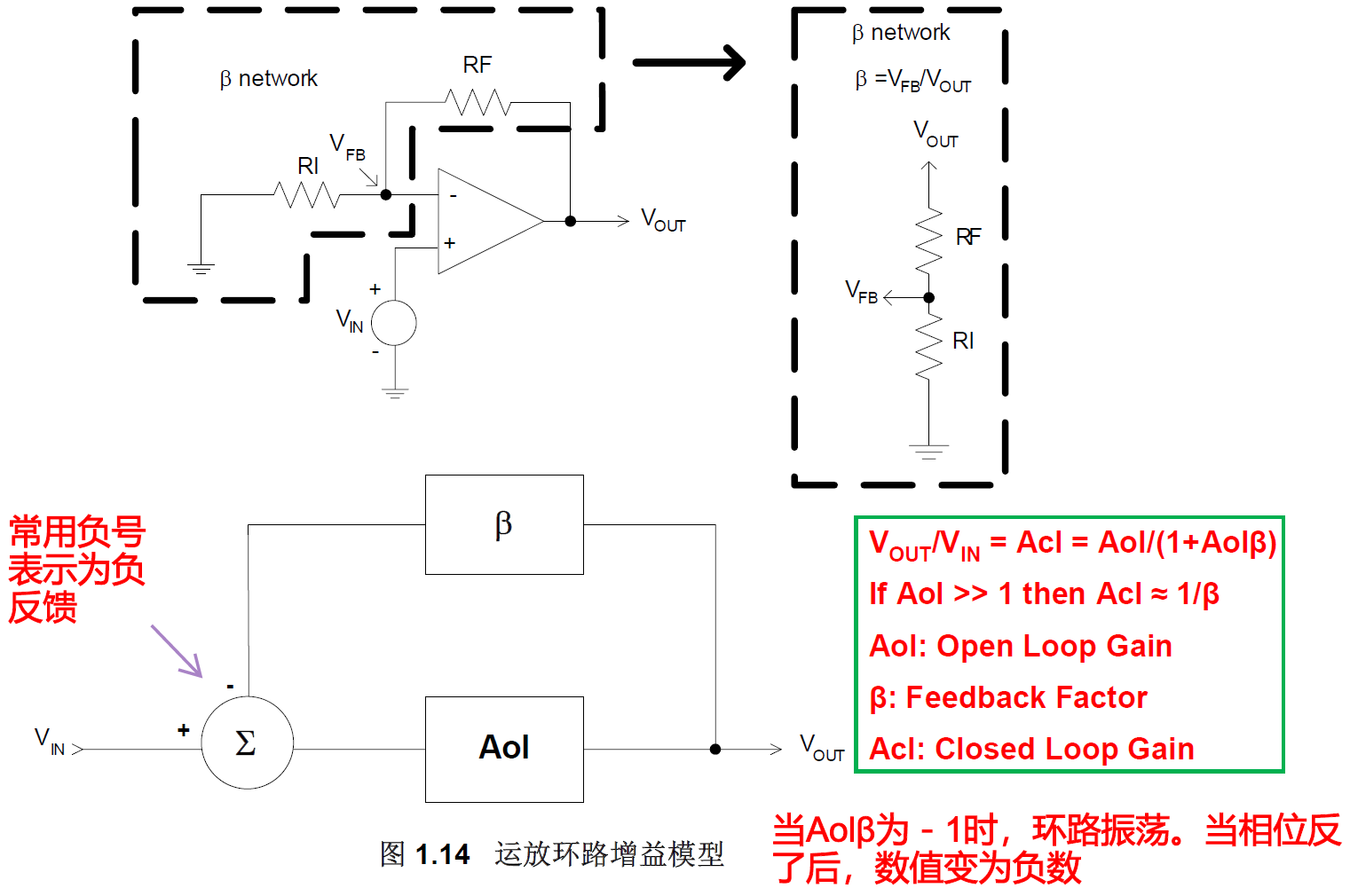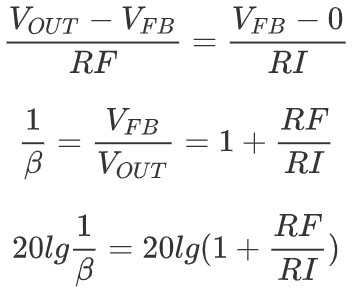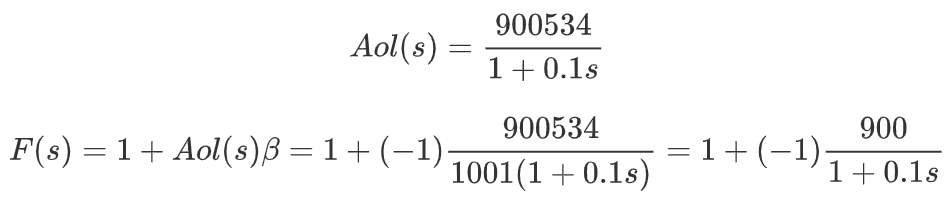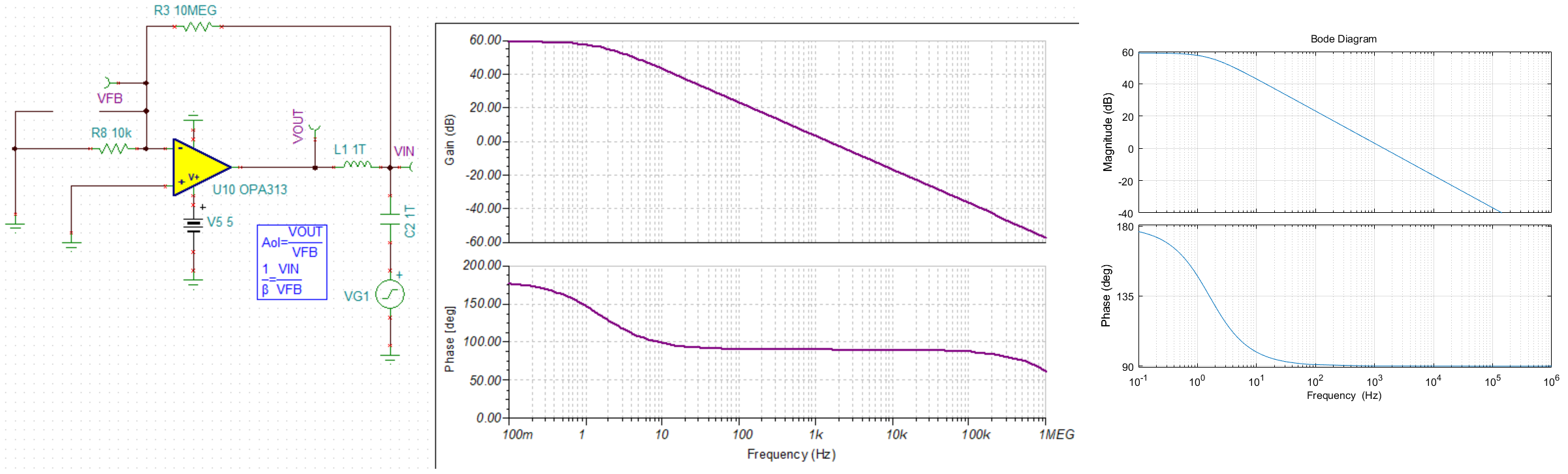负反馈Aolbeita曲线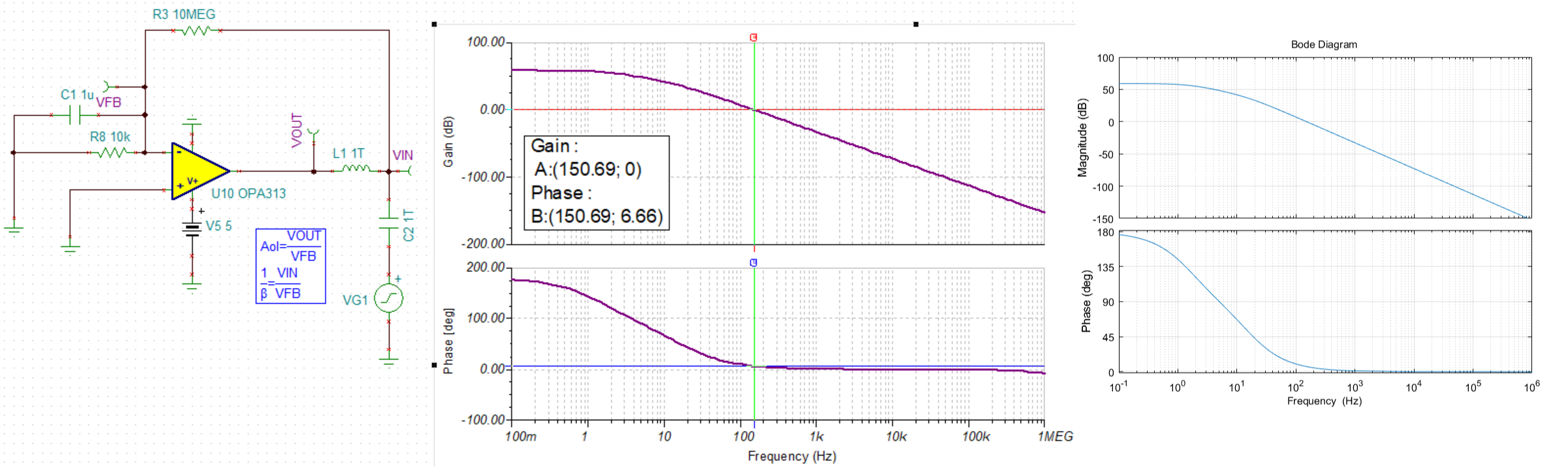负反馈Aolbeita传递函数加极点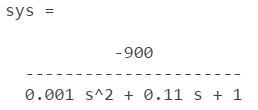负反馈Aolbeita-传递函数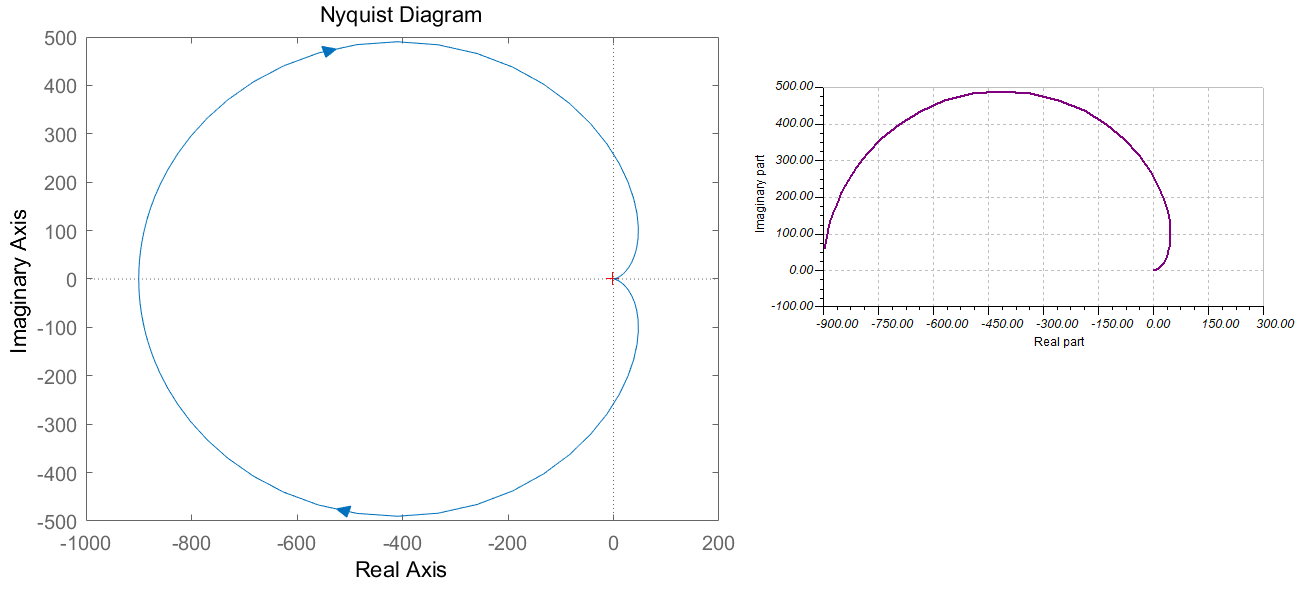负反馈Aolbeita-Nyquist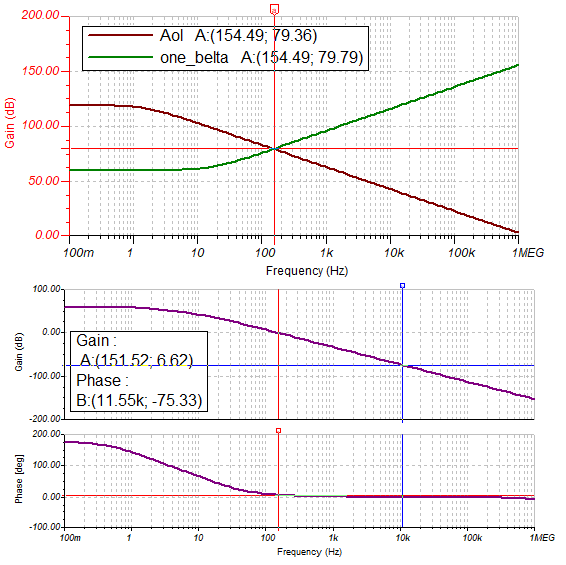负反馈Aolbeita-Aol-1_B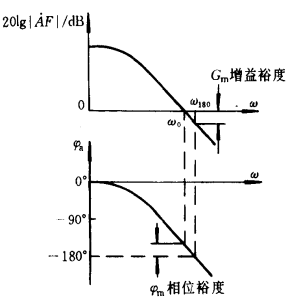增益裕度和相位裕度

```对于小信号的放大，一般认为在引入深度负反馈的情况下，在整个信号带宽内运放环路稳定，则电路设计没有问题。

（即噪声增益曲线，取决于反馈环路的设计）的交点判断，该交点决定了所能放大信号带宽,即闭环带宽fcl。
```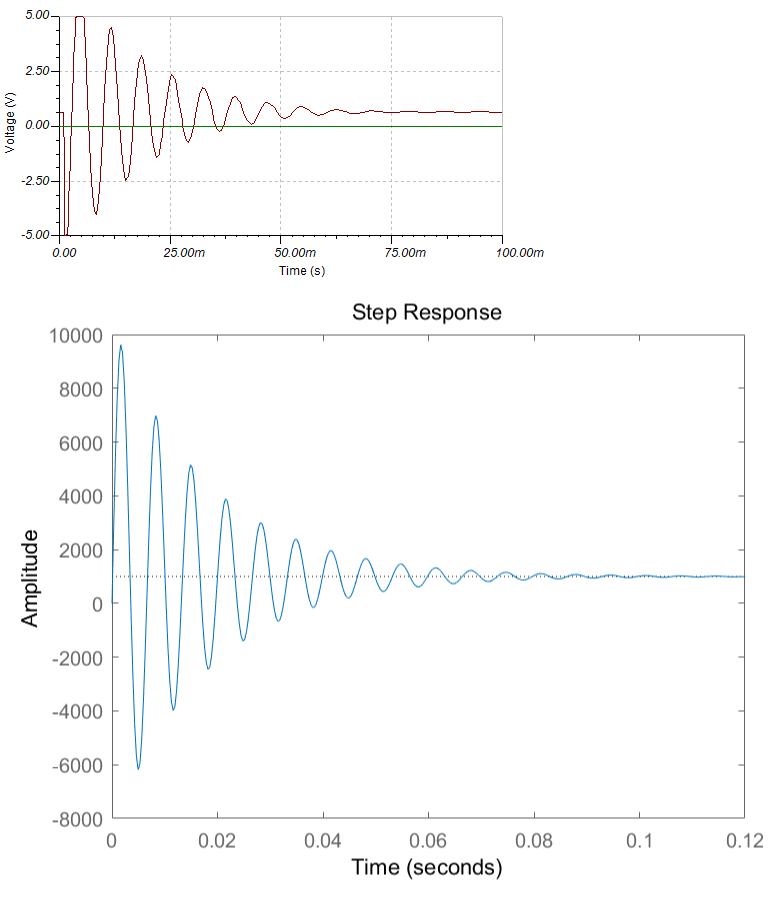## 环路增益测试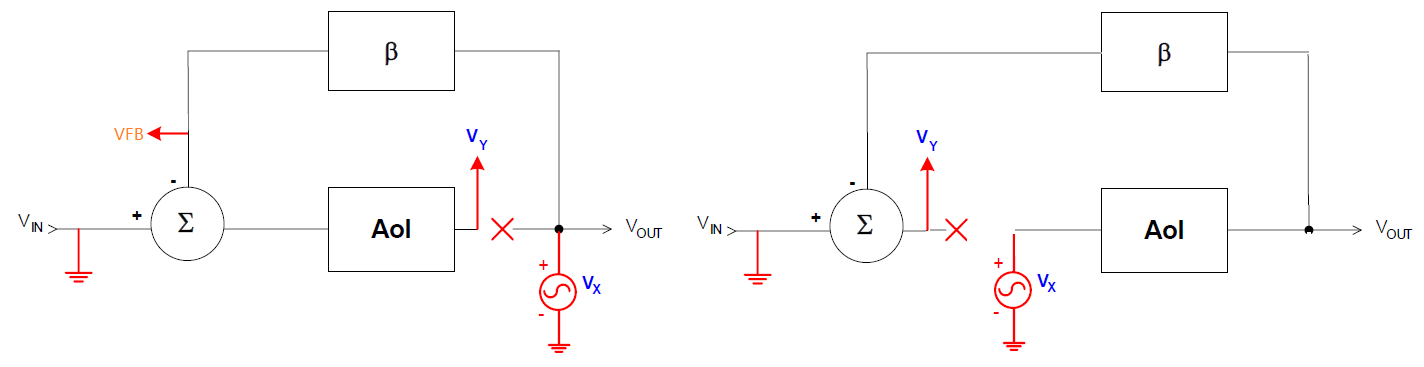环路增益测试结构

• 左边：Aol=Vy/VFB，1/β=Vx/VFB

• 右边：Aol=Vout/Vx，1/β=Vout/Vy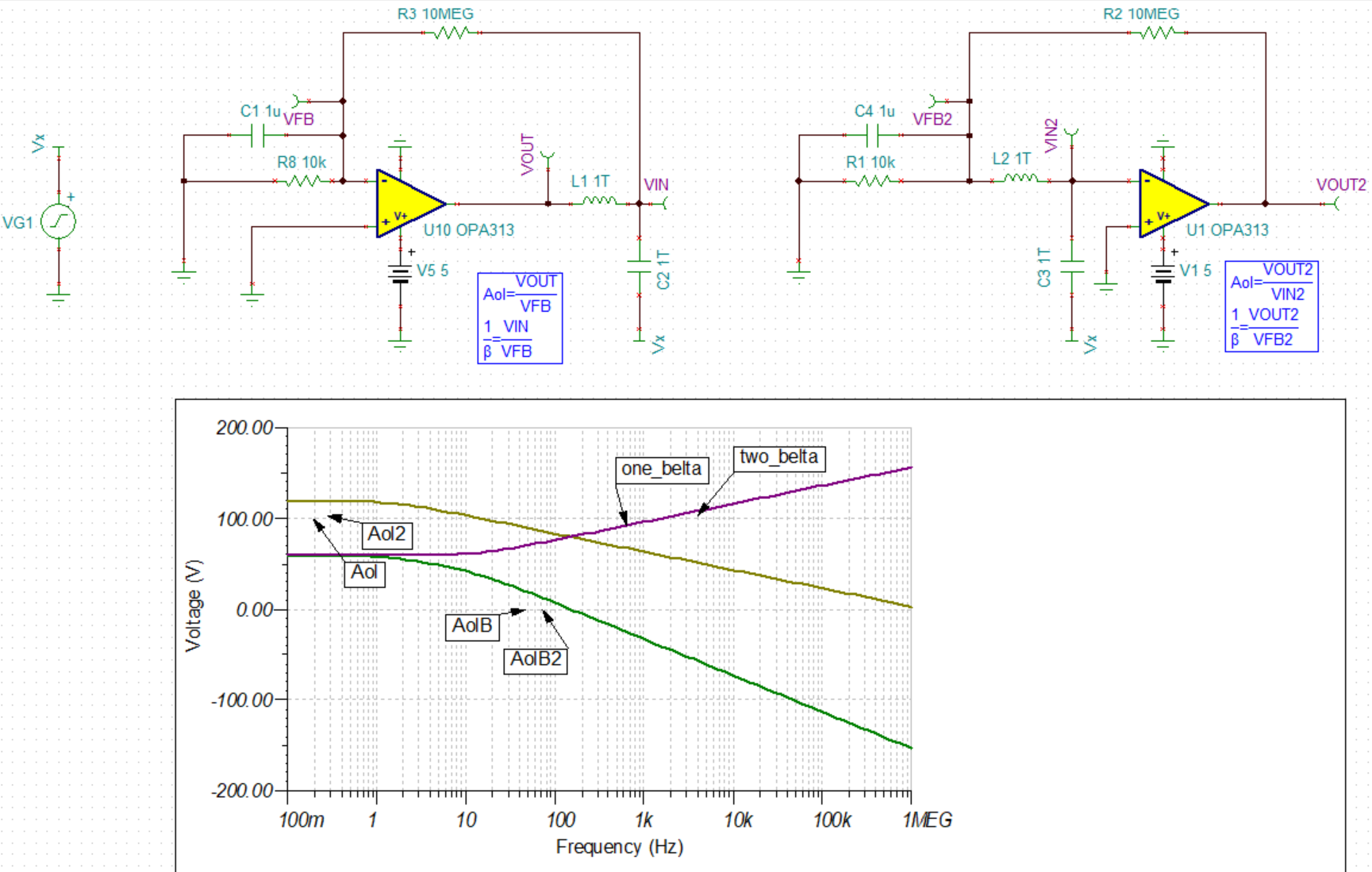环路增益测试仿真结果